# Mathematical morphology

(diff) ← Older revision | Latest revision (diff) | Newer revision → (diff)

A branch in digital image processing based on set-theoretical, geometrical and topological concepts, and as such particularly useful for the analysis of geometrical structure in an image. It has been applied in various disciplines, such as mineralogy, medical diagnostics, histology, remote sensing, pattern recognition, computer vision, etc. To a large extent, the current status is due to its founders G. Matheron [a1] and J. Serra [a2], [a3]. The first reference contains a comprehensive discussion on random sets and integral geometry. Actually, it is this probabilistic branch which has made morphology into such a powerful methodology. Other main references are [a4], [a5].

The central idea of mathematical morphology is to examine the geometrical structure of an image by matching it with small patterns at various locations in the image. By varying the size and the shape of the matching patterns, called structuring elements, one can extract useful information about the shape of the different parts of the image and their interrelations. In general, this procedure results in non-linear image operators, some of which will be described briefly below.

Originally, mathematical morphology has been developed for binary images; these can be represented mathematically as sets. The corresponding morphological operators use essentially three ingredients from set theory, namely set intersection, union, complementation. In the Euclidean case, translation is added to these three. Let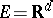(continuous case) or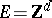(discrete case). Two basic morphological operators are dilationand erosion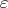, respectively given by Minkowski addition and subtraction: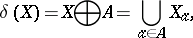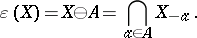Here,. The setrepresents the binary image andthe so-called structuring element. The choice of the structuring element depends on the application at hand.

It is obvious that dilation and erosion define increasing (or monotone) translation-invariant operators on. Every increasing translation-invariant operator can be written as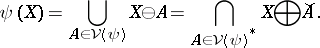Here,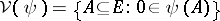is called the kernel of, and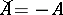. Furthermore,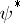denotes the negative operator given by, where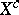is the complement of.

The composition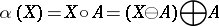is an idempotent (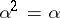), anti-extensive (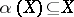, for every) operator. Operators with such properties are called openings. Dually, the composition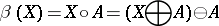is called a closing; it is idempotent and extensive (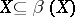, for every).

Openings and closings are used for many different tasks. First, they can be used for image restoration (e.g., noise filtering). Towards that goal, one generally builds compositions of openings and closings, called alternating sequential filters. Secondly, these operators can be used to compute the so-called pattern spectrum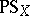of an image: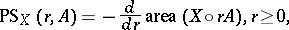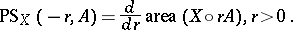Here,has to be a convex set. This pattern spectrum yields information about shape and/or texture of images: see [a6].

Some other important morphological transformations are the distance transform, the skeleton (or medial axis), geodesic reconstruction, and the hit-or-miss transform. Most of these tools were originally developed for binary images. Later, most of them have been extended for grey-scale images. Nowadays (1996), it is generally accepted that complete lattices constitute a natural theoretical framework for morphological operators (cf. Complete lattice). A comprehensive discussion of this framework is given in [a4].

How to Cite This Entry:
Mathematical morphology. Encyclopedia of Mathematics. URL: http://encyclopediaofmath.org/index.php?title=Mathematical_morphology&oldid=14030
This article was adapted from an original article by H.J.A.M. Heijmans (originator), which appeared in Encyclopedia of Mathematics - ISBN 1402006098. See original article